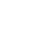< img height="1" width="1" style="display:none" src="https://www.facebook.com/tr?id=364346675014377&ev=PageView&noscript=1" />• Home
• Instant Online Quote• PCB Assembly
• PCB Capabilities
• Feedback
• Resources• About Us### Definition & Types & Factors of Characteristic Impedance

1. Definition of Characteristic Impedance

Characteristic Impedance (usually written as Z0) is the resistance in parallel circuits and power planes to the flow of alternating current. The characteristic impedance of a uniform transmission line is the ratio of the amplitudes of voltage and current of a single wave propagating along the line. Characteristic impedance is determined by the geometry and materials of the transmission line. The unit of characteristic impedance is the ohm.

Characteristic Impedance

2. Types of Characteristic Impedance

Currently the Characteristic Impedance is divided into Singleended impedance and Differential Impedance. Differential Impedance is divided into Odd mode, Even mode and Common mode. Characteristic impedance is also divided into Microstrip and Stripline.

3. Factors of Characteristic Impedance

The most critical characteristic impedance factors are the width of circuit trace, thickness of circuit trace, dielectric thickness-thickness of core, prepreg or solder mask around the circuit trace. Dielectric thickness is a function of core/prepreg selection and lamination parameters. Controlling the dielectric thickness relies on the layer configuration, the copper percentage of that layer, and the copper thickness. Line width is a result of plotting, imaging, developing, and etching. Soldermask will be applied on most PCB circuit boards as a protection layer. In almost all cases, soldermask will exist over the impedance traces on microstrip designs.

The following factors will also affect the impedance quality: model reliability, measurement integrity, process control and capability, defined work instructions, lab analysis. What’s more, these factors should also be taken into account: the dielectric constant of the material, soldermask thickness and its Dk value.